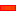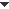﻿ Dialog: Blend-Curve

# Dialog: Blend-Curve

## Blend-Curve dialog-box

A Blend-Curve is a sketch-element with geometric-properties of position, angle, curvature and curvature-rate at its start-Point and its end-Point.

#### Geometric Properties of a Blend-Curve sketch-element

The geometric-properties of the Blend-Curve at its start-Point and its end-Point are its:

 • Position [edit with the Part-Editor in the normal way.]
 • Angle
 • Curvature
 • Curvature Rate
 • Velocity-Scale

If you merge the Blend-Point with other sketch-elements, the geometric-properties automatically become the same geometric-properties as the adjacent sketch-elements, in the default case.

You can also edit the geometric-properties to be different to the adjacent sketch-elements.

If can edit the geometric-properties:

 • exactly : with the Blend-Curve dialog-box
 • approximately : with the Blend-Curve drag-handles.

Edit the Geometric-Properties of a Blend-Curve

####EXACT EDITING: Blend-Curve dialog box

The two separators in the Blend-Curve dialog-box are the:

 • Start-Point parameters
 • End-Point parameters

The parameters in each separator are identicalStart Point [ or End Point ]

Angle [Units: º]:

Maximum = 360º ; Minimum = –360º

Blend check-box:

 ☑ [DEFAULT] The angle of the Blend-Curve becomes equal to the angle of the adjacent sketch-element at its start-Point (or end-Point)
 ❑ Enter the angle for the Blend-Curve at its start-Point (or end-Point).

Curvature k [Units:1 ∕ meters]:Notes on curvature

All shapes, squiggles, doodles, geometric shapes are a 'curve'. All points along a curve have a radius, its 'instantaneous radius'. We call this the curve's 'Radius-of-Curvature'. I prefer to call it the 'Radius of the Curve' as that is a more literal term.

When we draw a circle that:

 • is tangential to the curve
 • has a radius equal to the curve,
 • centre is on the normal of the curve.

we call the circle the Osculating Circle of the curve at that particular point along the curve. In general, the radius of the osculating-circle continually changes as it moves along the curve, and will also flip sides of the curve.

Curvature = 1Radius of the Osculating-Circle, or more generally, Curvature = 1/Radius-of-Curvature

Thus, as the radius of the osculating-circle decreases, the Curvature increases.

Curvature of a Line = 0, Curvature of a Circle = 1 ∕ Circle Radius.

E.g. : A circle has a radius of 20mm, its curvature = 1/0.02m = 50/m.

Blend check-box:

 ☑ [DEFAULT] The curvature of the Blend-Curve becomes equal to the curvature of the adjacent sketch-element, at its start-Point (or end-Point)
 ❑ Enter the curvature for the Blend-Curve its start-Point (or end-Point).

Curvature-Rate dk ∕ ds [Units: 1 ∕ m^2]:

Notes on Curvature Rate:

 Curvature-Rate is the rate-of-change of curvature [how rapidly curvature changes] with respect to the distance along the Blend-Curve. Only a different Blend-Curve can have a Curvature-Rate that is not zero. The Curvature-Rate of a Line and Arc are zero.

Blend check-box:

 ☑ Make the curvature-rate of the Blend-Curve equal the curvature-rate of the sketch-element with which you merge the Blend-Curve.at the start-Point (or end-Point)
 ❑ Enter a curvature-rate for the Blend-Curve that is different to the curvature-rate of the adjacent sketch-element at its start-Point (or end-Point).

Velocity Scaling

Increase Velocity-Scaling to 'inflate' the Blend-Curve.

Decrease Velocity-Scaling to 'deflate' the Blend-Curve.

Velocity Scaling parameter does not have units.

Default Value = 1 ; Maximum Value = 10 ; Minimum 0.01

####Approximate Editing: Blend-Curve Drag-HandlesTo edit the properties of the Blend-Curve with the Drag-Handles:

 1 Click the Blend-Curve one time to show Drag-Handles (one click) - see mouse-pointer in image to the left

Four Green drag-handles show - two at the start-Point and two at the end-Point - but they are not active.

At each end of the Blend-Curve, there is a drag-handle with an:

 • Arrow: this drag-handle can control the Velocity-Scaling and the Angle
 • Small Circle: this drag-handle can control the Curvature.

You must use the Blend-Curve dialog to control the Curvature-Rate.To make a Drag-Handle Active

You cannot drag a Green Drag-Handle.

 1 Double-click a drag-handle to make it active

You must double-click each drag-handle to make each one active.

The colour of the drag-handle will change to Blue to indicate the drag-handle is 'active'.To change the angle of the Blend-Curve at the start-Point or end-Point , and also to change the overall length of the Blend-Curve

 1 Drag the drag-handleYou can drag the handle in two ways.

 a) Move the arrow head further away from the Point to increase the Velocity-Scaling factor
 b) Rotate the arrowhead around the Point to control its Angle.

If you rotate the arrow-head around the Point, the Angle 'blend' with the adjacent sketch-element is broken.To change the curvature of the Blend-Curve at the start-Point or end-Point or both:

If you move the Small-Circlebetween 90º and ±180º

 • The Curvature increases from 0 Curvature (a straight-line) to +ve 'Large Value'

If you move the Small-Circlebetween 90º and ±180º

 • The Curvature increases from 0 (a straight-line) to –ve 'Large Value'

Note: the angle is always relative to the +Y-axis of the Mechanism Plane, Not the Angle of the Blend-Curve.

Thus 0º is Vertical Upwards - always- and will tend to make the Blend-Curve 'straight' at the Point.

#### Smoothness and Geometric-Properties of sketch-elements

You frequently need a sketch to be smooth when it acts as the Path for a Motion-Point.

To join Blend-Curves to other sketch-elements, and other Blend-Curves, and to keep the sketch-path smooth:

Imagine that one Blend-Curve is 'later' or 'after' another:

 1 Edit the Blend-Curve that comes later
 2 Select the start-Point separator in the Blend Curve dialog
 3 Click all of the 'Blend' check boxes
 4 Edit the Blend-Curve that comes earlier
 5 Select the end-Point separator in the Blend-Curve dialog
 6 Clear the 'Blend' check boxes

Now edit the values of the end-Point.

 7 Edit the values of the end-Point of the 'earlier' Blend-Curve

As you edit the values, the start-Point of the Blend-Curve that is 'later' will update to the new values you enter in the 'earlier' Blend-Curve.

Tutorial and Reference Help Files for MechDesigner and MotionDesigner 13.2 + © Machine, Mechanism, Motion and Cam Design Software by PSMotion Ltd